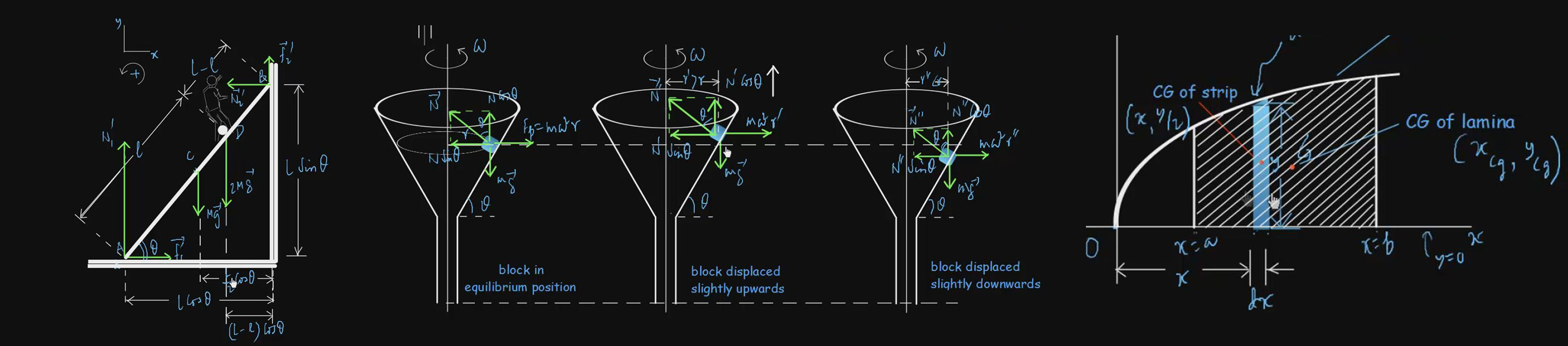# Circular Motion

In the present topic, the same motion will be discussed in greater detail. The topic deals with the kinematics of circular motion without any reference to the forces that cause it as well as the contribution of the forces acting on a particle, causing its circular motion. The study of circular motion is not only important in itself, but also an essential precondition for the study of rotational motion. When a rigid body rotates about an axis, every particle of it describes a circle whose centre lies on the axis of rotation. Therefore, the kinematic equations that we develop in this topic will also be useful to study rotational motion. This topic consists of video lectures on Angular Quantities in Circular Motion, Circular Motion with Constant Angular Acceleration, Two Accelerations of Non-Uniform Circular Motion, Problems on Circular Motion and many more to provide a thorough knowledge and to guide students to develop the problem-solving skill.

#### Basic level videos

Angular Quantities In Circular Motion 1:11:16 Basic
350 7.5
Circular Motion With Constant Angular Acceleration 31:51 Basic
200 3
Derivation Of Centripetal Acceleration 42:19 Basic
250 4
Examples Of Nonuniform Circular Motion 1:04:17 Basic
350 7.5
Examples Of Uniform Circular Motion 42:49 Basic
250 4
Examples Of Uniform Circular Motion II 47:42 Basic
300 5
Problems On Circular Motion 33:45 Basic
200 3
Problems On Circular Motion II 57:52 Basic
300 5
Problems On Circular Motion III 34:51 Basic
200 3
Problems On Circular Motion With Constant Angular Acceleration 32:47 Basic
200 3
Relations Between Linear And Angular Quantities 38:28 Basic
200 3
Two Accelerations Of Nonuniform Circular Motion 53:04 Basic
300 7.5

#### Advanced level Videos Note: (CE) Stands for Problems from Competitive Examination Papers

Advanced-Level Problems On Circular Motion I 1:03:41
350
7.5
Advanced-Level Problems On Circular Motion III 1:18:10
350
7.5
Advanced-Level Problems On Circular Motion IV 1:17:02
350
7.5